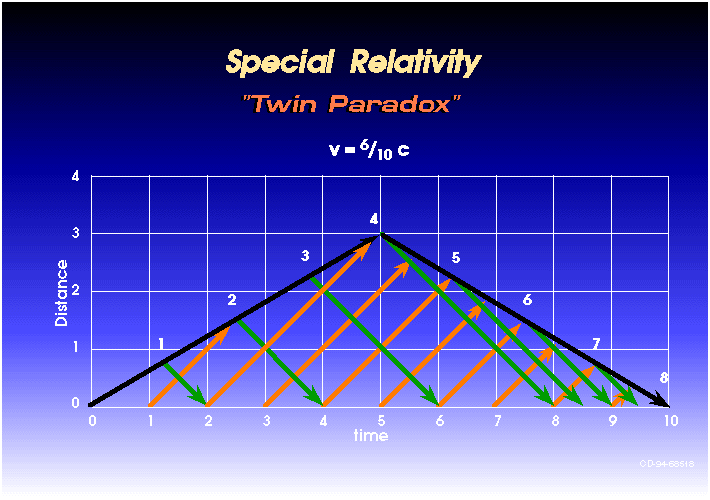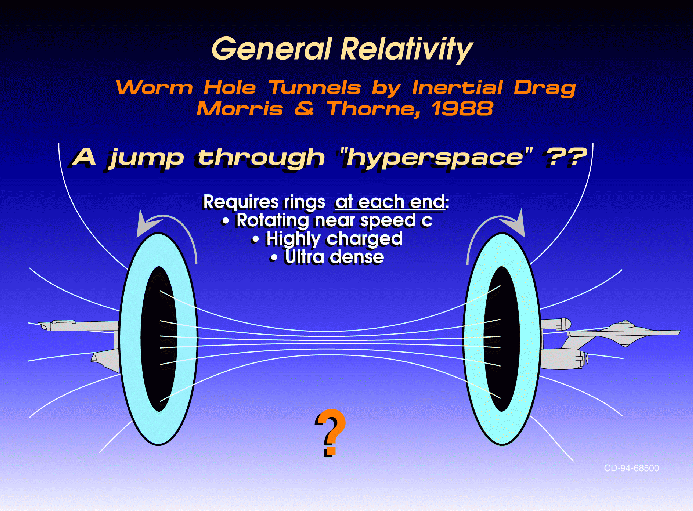There are many theories that relate between physics and astronomy. One theory refers to the“physical theory of measurement” and thus explains the speed of light, the movement of different bodies and objects, and also of uniform motion. This is none other than the theory of Special Relativity.

The term Special Relativity refers to the theory established by the famous physicist and the father of relative physics, Albert Einstein in the year 1905. The said theory also simplifies the old theory of Galileo Galilei on his principles of uniform motion—that in a certain constant motion of a certain object, there is no definite or absolute zero acceleration or state of rest.

The theory also incorporates the different topics of mechanics, the physical laws, and also the laws concerning both mechanics and electrodynamics. This theory established has been verified countless numbers of times, even in countermeasures. It also passed the different topics like the length contraction, time dilation and even the simultaneity relativity principles. It also contradicts the idea that the time interval duration of the events is equal for all the observers.The said theory also contains Einstein’s famous equation, the formula for the speed of light, wherein the energy is equal to the mass and the speed of light squared, also keeping in mind that the speed of light is the form of a vacuum. It also complies with the Newtonian Mechanics in terms of application, and it also introduces the concept of space time, wherein it is the dimension where both time and space join as one continuum.